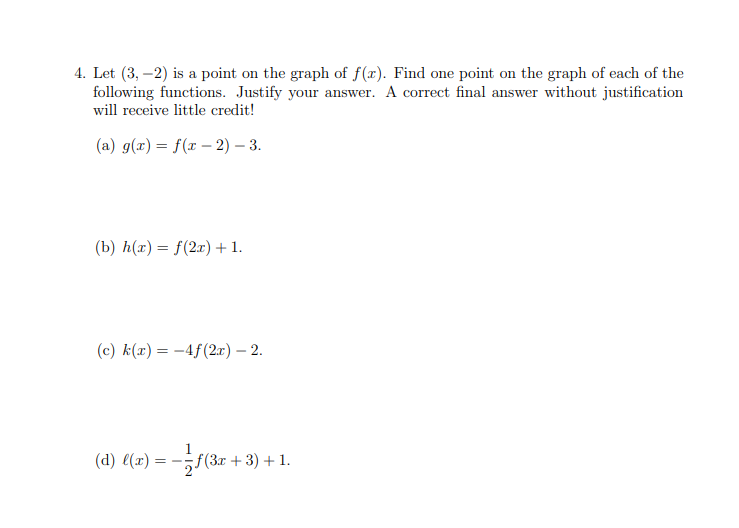# 4. Let (3, -2) is a point on the graph of f(x). Find one point on the graph of each of the following functions. Justify your answer. A correct final answer without justification will receive little credit! (a) g(r) f(x- 2) 3. (b) h(") %—D f(2л) + 1. (c) k(x) 4f(2x) - 2. (d) l(x)= {(3г + 3) + 1.

Question

Practice Question 4.3help_outlineImage Transcriptionclose4. Let (3, -2) is a point on the graph of f(x). Find one point on the graph of each of the following functions. Justify your answer. A correct final answer without justification will receive little credit! (a) g(r) f(x- 2) 3. (b) h(") %—D f(2л) + 1. (c) k(x) 4f(2x) - 2. (d) l(x)= {(3г + 3) + 1. fullscreen

### Want to see the step-by-step answer?